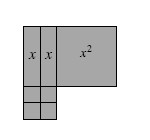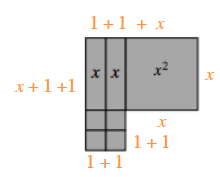### Home > AC > Chapter 2 > Lesson 2.1.4 > Problem2-45

2-45.

Write an expression that represents the perimeter of the shape built with algebra tiles below.Perimeter is the distance around a shape.

Find the length of all the sides by adding the individual parts.

Add all the side lengths together and simplify.

$(x+1+1)+(1+1+x)+(1+1)+(1+1)+(x)+(x)=?$

$4x+8$